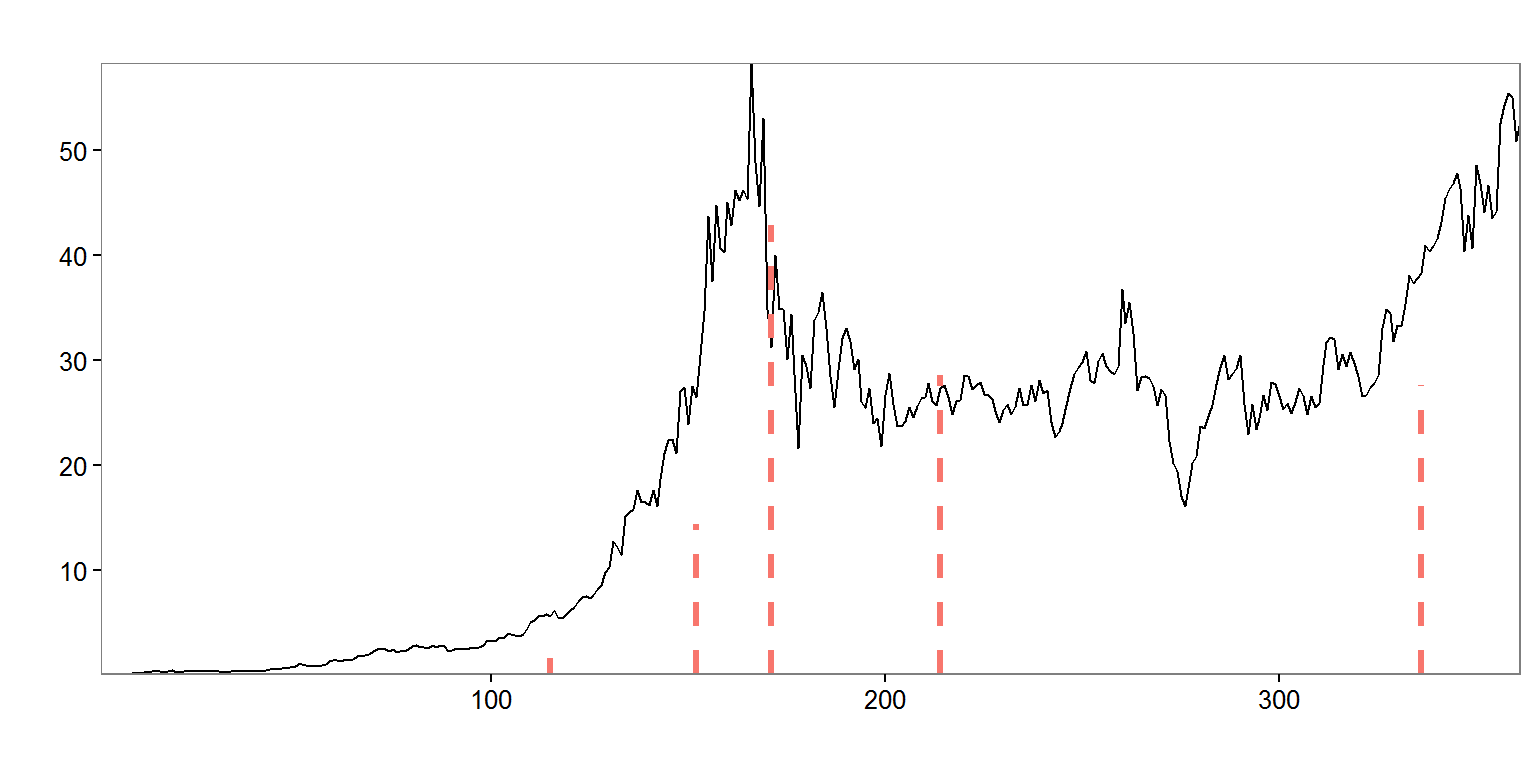March 10, 2016

## Introduction

• Background
• PhD in Bioinformatics, MS Biostatistics (U of M)
• Data Scientist at Trinity Health (1.5 years)
• Two boys (2.5 and 0.25 years)## Motivation

• Medical billing codes assigned to encounter
• Aggregate code usage (monthly)
• "Did we observe more live births at SJMHS than usual in November?"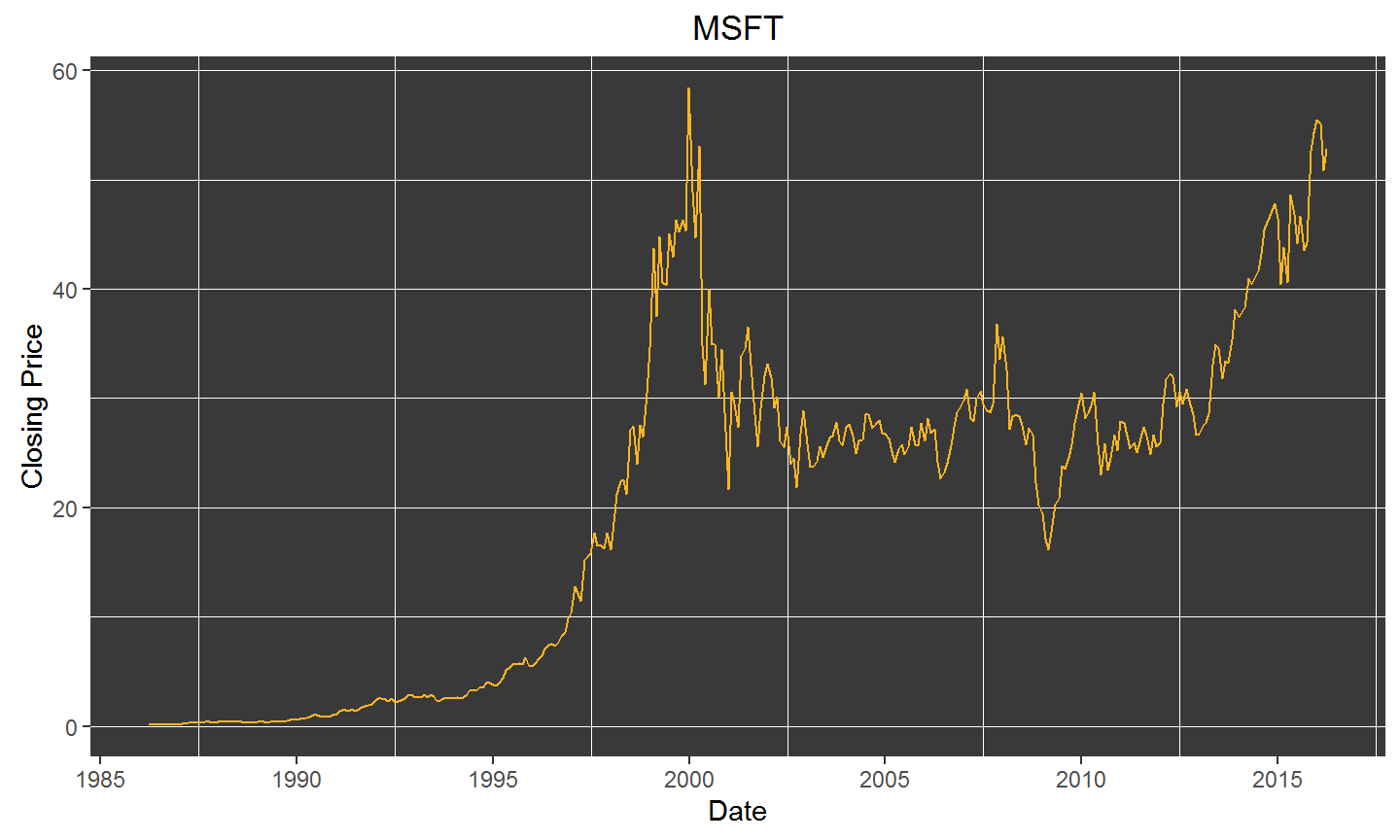## Decomposition of time series

STL: a seasonal-trend decomposition procedure based on LOESS
Robert B. Cleveland, William S. Cleveland, Jean E. McRae, and Irma Terpenning
Journal of Official Statistics (1990)

• Trend component: low-frequency variation and long-term changes in data
• Seasonal component: seasonal variation in data
• Remainder component: variation in data without trend and seasonal components

For $$\nu$$ in $$1\dots N$$,

$Y_\nu = T_\nu + S_\nu + R_\nu$

## LOESS fit poorly approximates underlying trend

msft_data %>%
ggplot(aes(x = timestamp, y = count)) +
geom_point() +
geom_smooth(method = "loess", color = "red") +
xlab("Date") +
ylab("Closing Price") +
ggtitle("MSFT")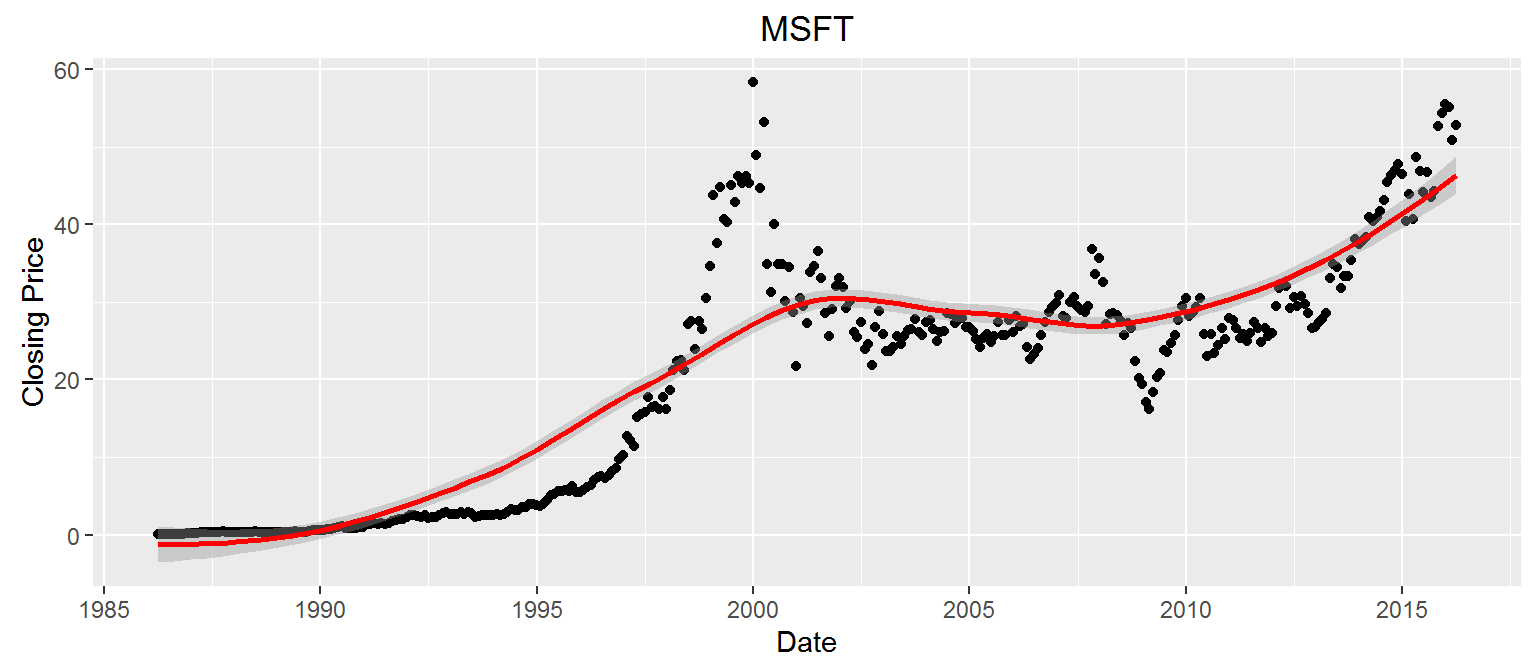## STL to separate time series components

msft_zoo <- zoo::zoo(msft_data$count, order.by = msft_data$timestamp) # i like zoo, so sue me
msft_ts <- stats::ts(msft_zoo, frequency = 12)                        # monthly data, so 12 obs./period
msft_stl <- stats::stl(msft_ts, s.window = "periodic")                # seasonal decomposition by loess
plot(msft_stl)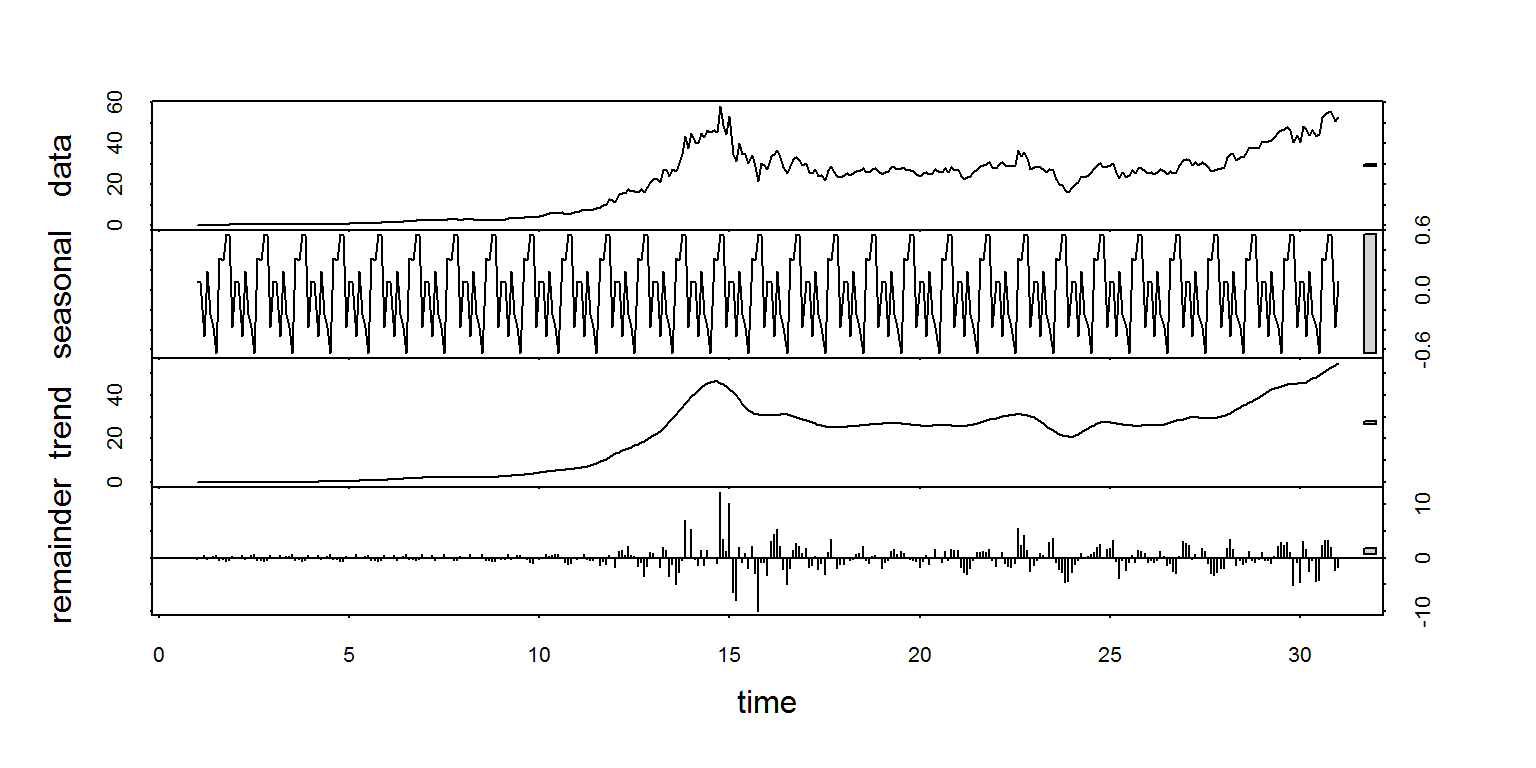## Increased robustness if outliers are expected

msft_stl <- stats::stl(msft_ts, s.window = "periodic",
s.degree = 1,                 # seasonal polynomial fit degree, {0, 1}
robust = T)                   # robust fitting
#                      inner = if(robust)  1 else 2, # backfitting iterations
#                      outer = if(robust) 15 else 0  # robustness iterations
plot(msft_stl)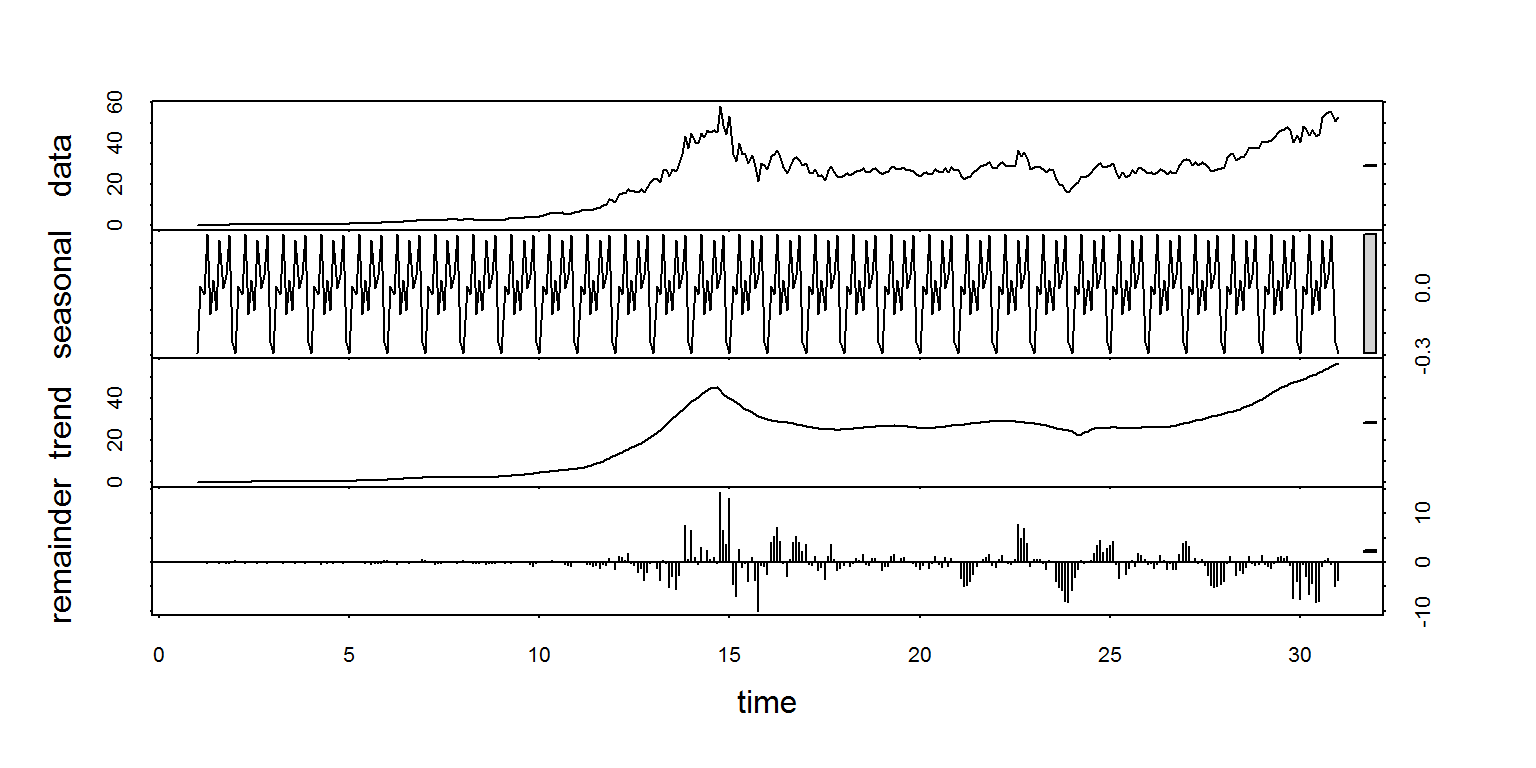## Outlier detection from univariate time series

• Residual component roughly follows a standard normal distribution
• Exploit this using Student's t-distribution
• Grubbs' test for outliers (extreme studentized deviate test, or ESD)
• Test is iterated until no outliers are found (e.g. reject $$H_0$$)

$G = \frac{\max\limits_{\nu}\left|R_\nu-\bar{R}\right|}{s}$

$$H_0$$: no outliers

$$H_a$$: at least one outlier

For two-sided test, the null hypothesis is rejected if

$G \gt \frac{N-1}{\sqrt{N}} \sqrt{\frac {t^2_{\alpha/(2N),N-2}} {N-2+t^2_{\alpha/(2N),N-2}} }$

## Extracting the residual component from STL

names(msft_stl)
##  "time.series" "weights"     "call"        "win"         "deg"
##  "jump"        "inner"       "outer"
head(msft_stl$time.series) ## seasonal trend remainder ## [1,] -0.292745057 0.1035591 0.289185945 ## [2,] 0.006206882 0.1111303 -0.007337161 ## [3,] -0.027554415 0.1187014 0.028852968 ## [4,] 0.238298734 0.1291275 -0.257426188 ## [5,] -0.117503871 0.1395535 0.077950408 ## [6,] 0.032527383 0.1519589 -0.084486241 ## Approximate normality of residual component residComp <- msft_stl$time.series[,3]
hist(residComp, breaks = 50, main = "", xlab = "Residual Component")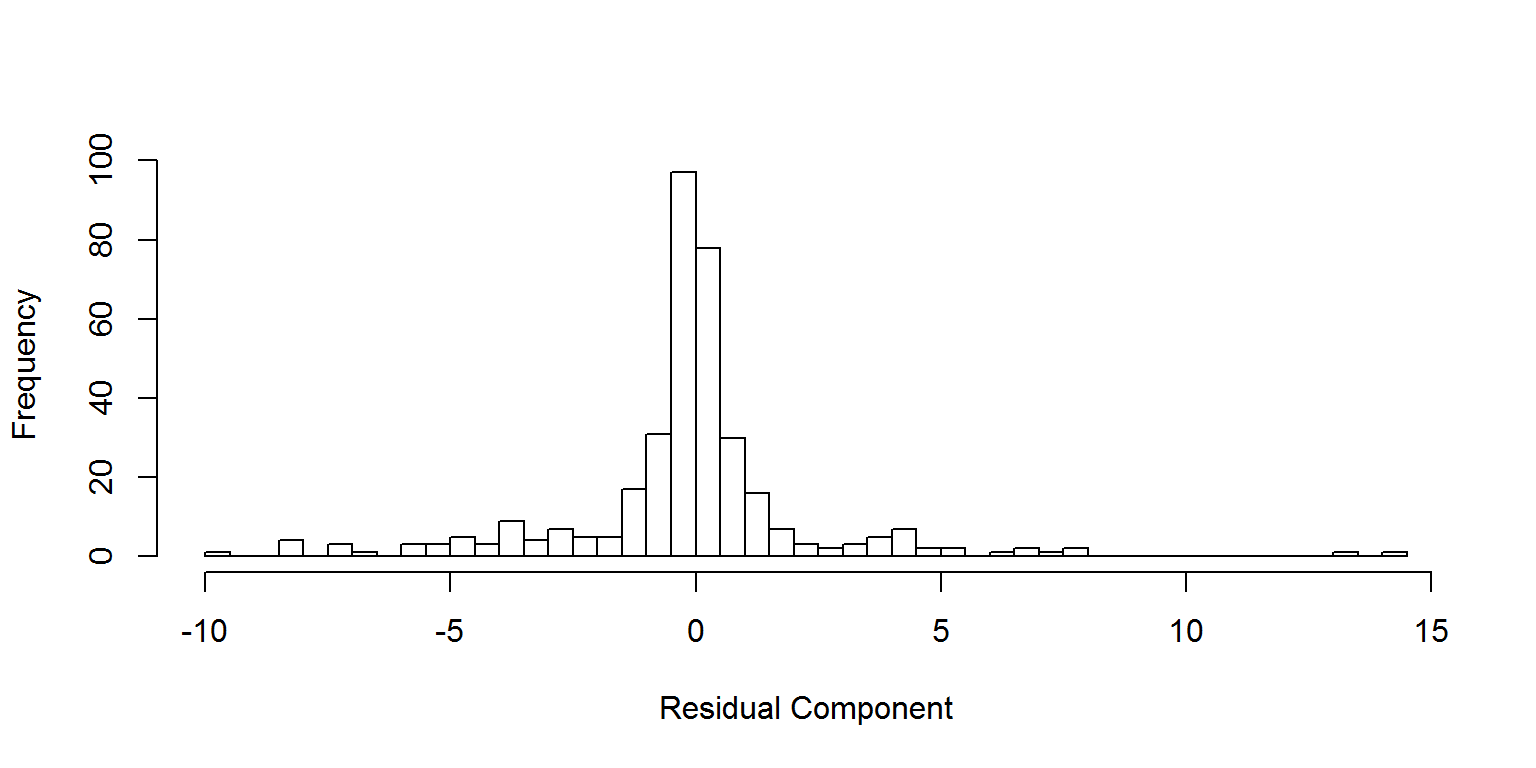• Designed to detect outliers in time series data
• Seasonal hybrid ESD (S-H-ESD)
• Uses piecewise median instead of STL trend
• More robust to extreme outliers
• $$Y_\nu$$ is split into non-overlapping windows

Residual component is calculated as:

$R_\nu = Y_\nu - S_\nu - \tilde{Y_\nu}$

Then ESD is calculated using this residual and median absolute deviation (MAD)

$G = \frac {\max\limits_{\nu} \left| R_\nu-\tilde{R} \right| } {median \left| R_\nu - \tilde{R} \right| }$

## AnomalyDetection syntax and results

msft_vec <- msft_data$count[order(msft_data$timestamp)]
outliers <- AnomalyDetectionVec(msft_vec, plot = T,
direction = 'both',   # detect positive and negative spikes
period = 12,          # number obs. in single period
longterm_period = 72) # number obs. for which trend is "flat"
outliers$plot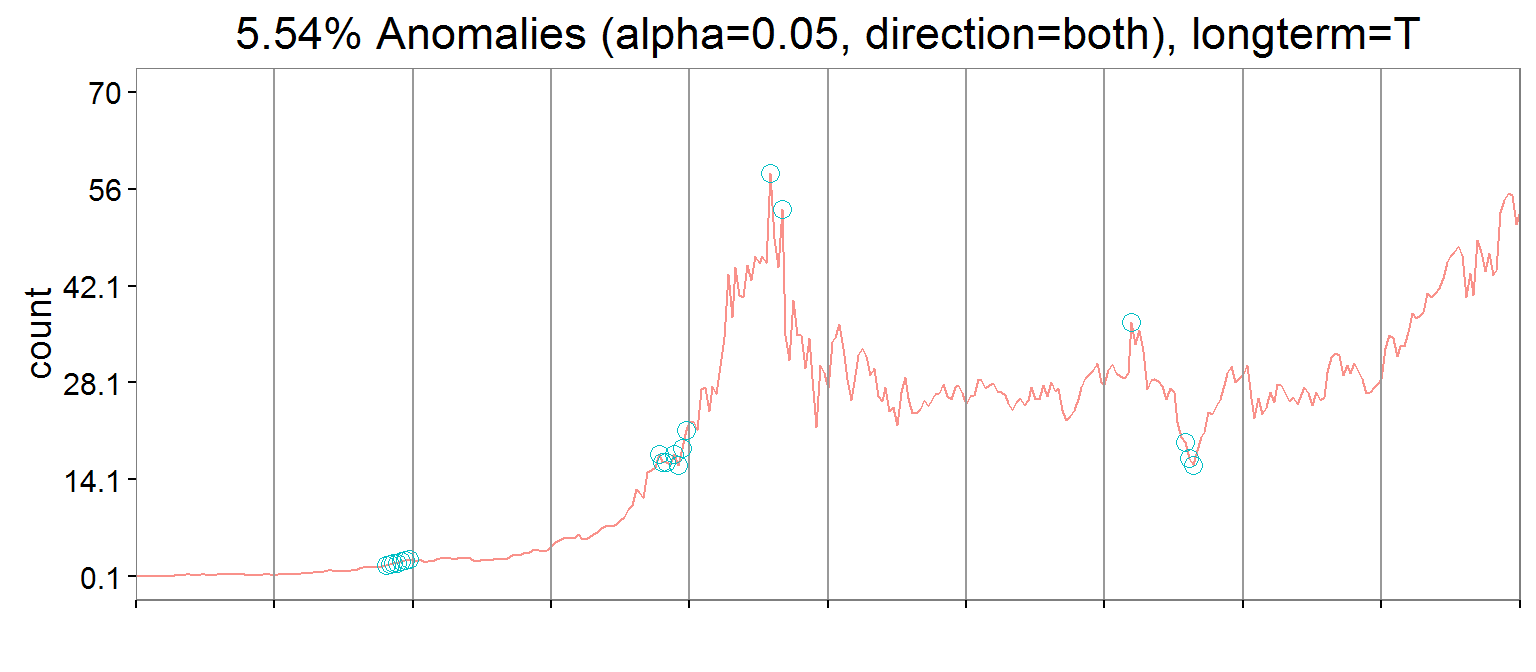## BreakoutDetection from Twitter • Designed to detect mean shifts in time series data • E-Divisive with Medians (EDM) • Robust to extreme outliers • Non-parametric For $$Y_1,\,Y_2,\,\dots,\,Y_N$$, let there be a point $$\tau$$ where $Y_1,\,Y_2,\,\dots,\,Y_\tau \thicksim F$ $Y_{\tau+1},\,Y_{\tau+2},\,\dots,\,Y_N \thicksim G$ Kolmogorov-Smirnov test to compare distributions $$H_0 \colon F = G$$ $$H_a \colon F \neq G$$ But we don't know the location and number of $$\tau$$… ## E-Divisive (with medians) A Nonparametric Approach for Multiple Change Point Analysis of Multivariate Data David S. MAtteson and Nicholas A. James arXiv (1990) • algorithms to narrow search space • Twitter's modification uses medians to estimate the energy distance between two distributions • Energy distance combines within and between levels of variation • Tree searching (bisection) limits the space in which this must be calculated ## BreakoutDetection syntax and results changes <- breakout(msft_vec, plot = T, method = 'multi', # detect multiple change points min.size = 12) # min(# obs.) between change points changes$plot